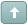Error

 Previous Topic Next Topic
 KTP2 #1 Posted : Tuesday, November 11, 2008 4:44:18 PM(UTC)Rank: Advanced MemberGroups: Registered, Registered Users, SubscribersJoined: 2/2/2007(UTC)Posts: 367Was thanked: 1 time(s) in 1 post(s) To create the Modified Moving Average, as explained in the article "Quicken Your Averages" by Joe Sharp, in MetaStock 6.52 or higher, select Indicator Builder from the Tools menu, click New and enter the following code, according to the desired period. Name: Modified Moving Average For a 2 period average/p> N:=2;TN:=Mov(C,N,S);s1:=((n-1)/2)*C+((n-3)/2)*Ref(C,-1); y2:=TN+(6*S1)/((n+1)*n); y2 For a 3 period average N:=3;TN:=Mov(C,N,S);s1:=((n-1)/2)*C+((n-3)/2)*Ref(C,-1)+((n-5)/2)*Ref(C,-2); y2:=TN+(6*S1)/((n+1)*n); y2 For a 4 period average N:=4;TN:=Mov(C,N,S);s1:=((n-1)/2)*C+((n-3)/2)*Ref(C,-1)+((n-5)/2)*Ref(C,-2)+((n-7)/2)*Ref(C,-3); y2:=TN+(6*S1)/((n+1)*n); y2 For a 10 period average N:=10;TN:=Mov(C,N,S);s1:=((n-1)/2)*C+((n-3)/2)*Ref(C,-1)+((n-5)/2)*Ref(C,-2)+((n-7)/2)*Ref(C,-3)+((n-9)/2)*Ref(C,-4)+((n-11)/2)*Ref(C,-5)+((n-13)/2)*Ref(C,-6)+((n-15)/2)*Ref(C,-7)+((n-17)/2)*Ref(C,-8)+((n-19)/2)*Ref(C,-9); y2:=TN+(6*S1)/((n+1)*n); y2Wanna join the discussion?! Login to your Discussions forum accountor Register a new forum account.Users browsing this topic
Forum Jump
You cannot post new topics in this forum.
You cannot reply to topics in this forum.
You cannot delete your posts in this forum.
You cannot edit your posts in this forum.
You cannot create polls in this forum.
You cannot vote in polls in this forum.